# The Calculator Encyclopedia Calculates and Solves the Wavelength for a Wave – Particle Behaviour

According to Wikipedia,

Wave–particle duality is the concept in quantum mechanics that every particle or quantum entity may be partly described in terms not only of particles, but also of waves.

In physics, the wavelength is the spatial period of a periodic wave—the distance over which the wave’s shape repeats.

Nickzom Calculator requires two parameters to compute the wavelength of a wave or particle. These parameters are:

• Mass
• Velocity

The formula for computing the wavelength is:

λ = h / mv

Where:
λ = Wavelength
h = Planck’s Constant (6.63 x 10-34 js)
m = Mass
v = Velocity

Let’s solve an example, find the wavelength of a wave – particle with a mass of 300 Kg and a velocity of 3 x 1010 m/s.

From the example, we can see that:
m = 300
v = 3 x 1010

λ = 6.63 x 10-34 / 300 (3 x 1010)
λ = 6.63 x 10-34 / 9 x 1012
λ = 7.37 x 10-47

Therefore, the wavelength of the wave – particle (λ) is 7.37 x 10-47.

Now, I am going to guide you through the process of entering your values into The Calculator Encyclopedia (Nickzom Calculator) to solve and present you with the formula, workings and answer.

To have access to the calculator encyclopedia – Nickzom Calculator click on any of these links that suits best:
Web – https://www.nickzom.org/calculator-plus
Apple (Paid) – https://itunes.apple.com/us/app/nickzom-calculator/id1331162702?mt=8

it is preferable you obtain the professional version because that is what we are using in Nickzom Blog to showcase the powers of the calculator encyclopedia. Once you have got access to the app, proceed to the Calculator Map, then click on Wave-Particle Behaviour under the Physics section.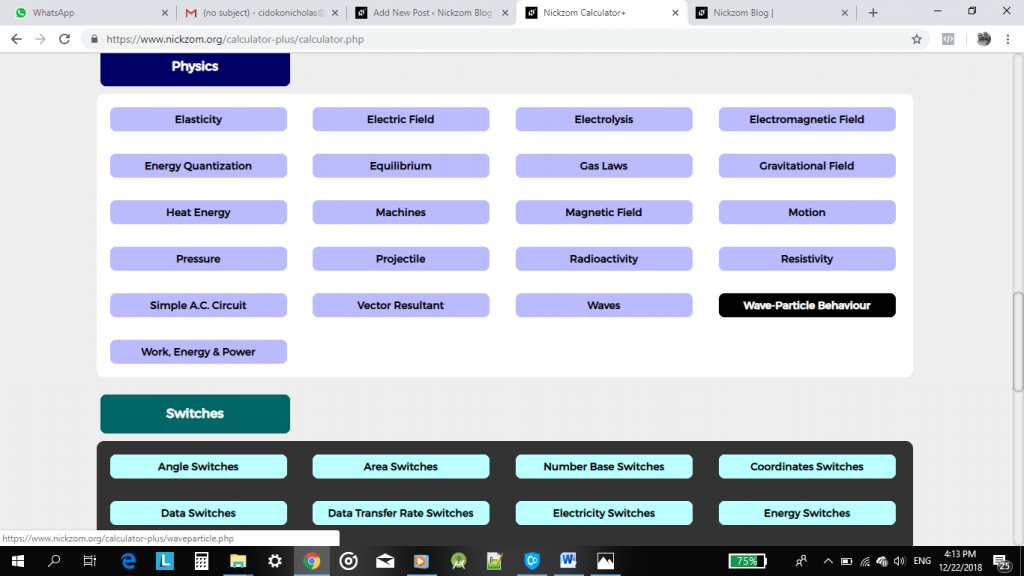Clicking on the Wave-Particle Behaviour would display the screenshot below for you to enter the values of the required parameters.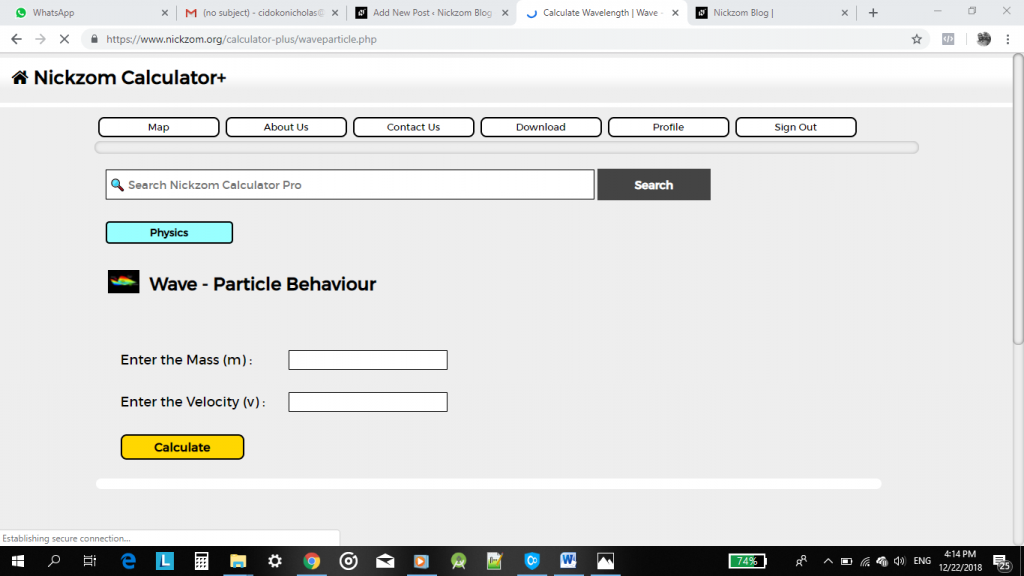To enter any value in standard or scientific format is represented by the letter E.
For Example: 3 x 1010 is entered as 3E10.

Now, enter 300 for mass and 3E10 for velocity.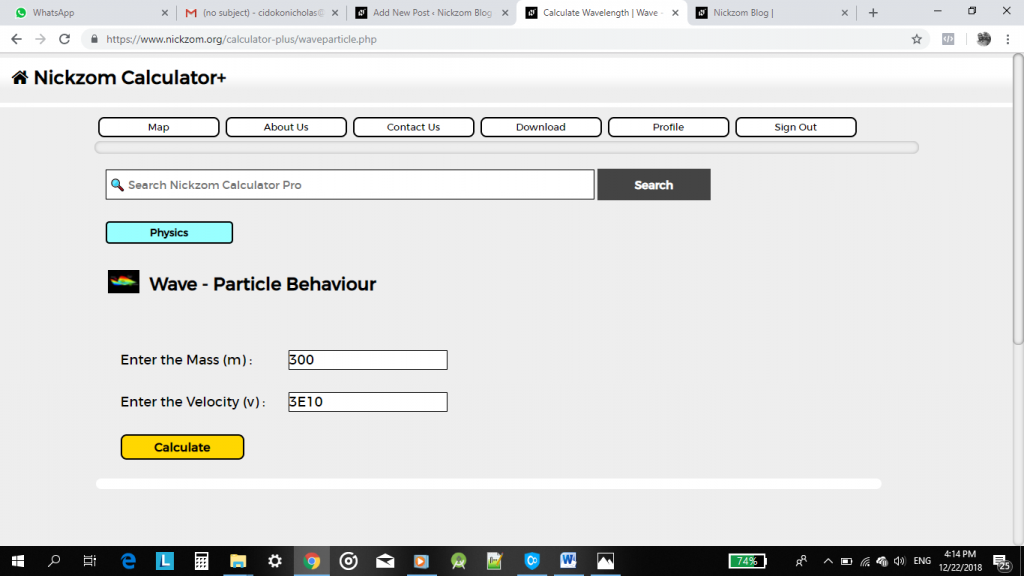Then, click on the Calculate button.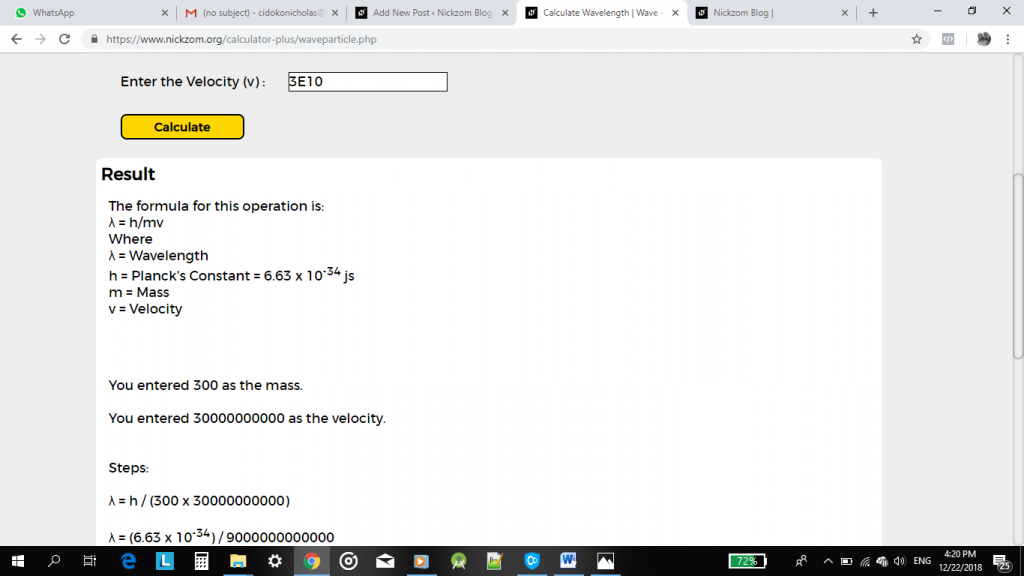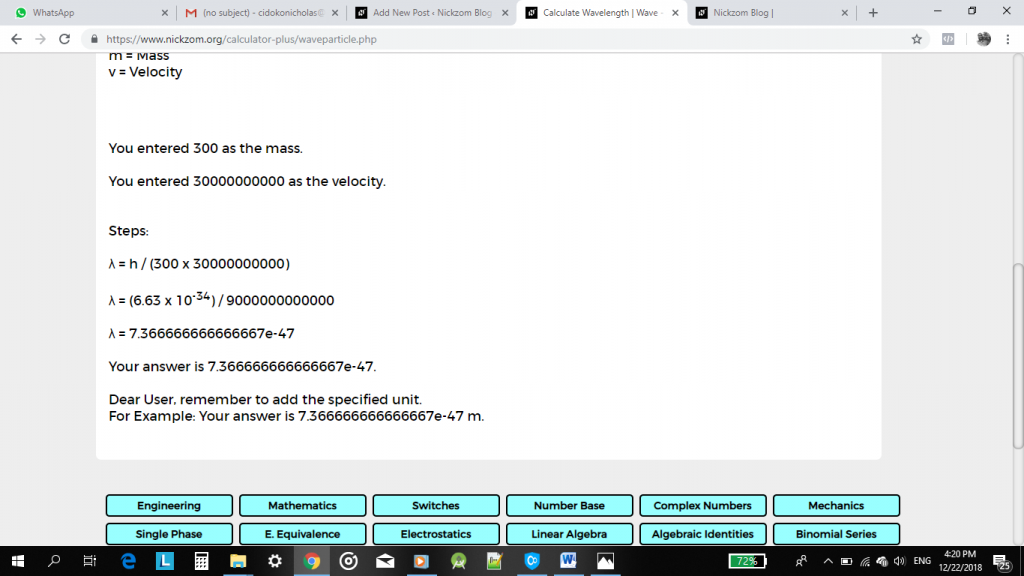You can see that you do not need to perform any calculation. All you need are the values and once you enter them and click on calculate, you get a full result with the formula, workings (steps) and answer.